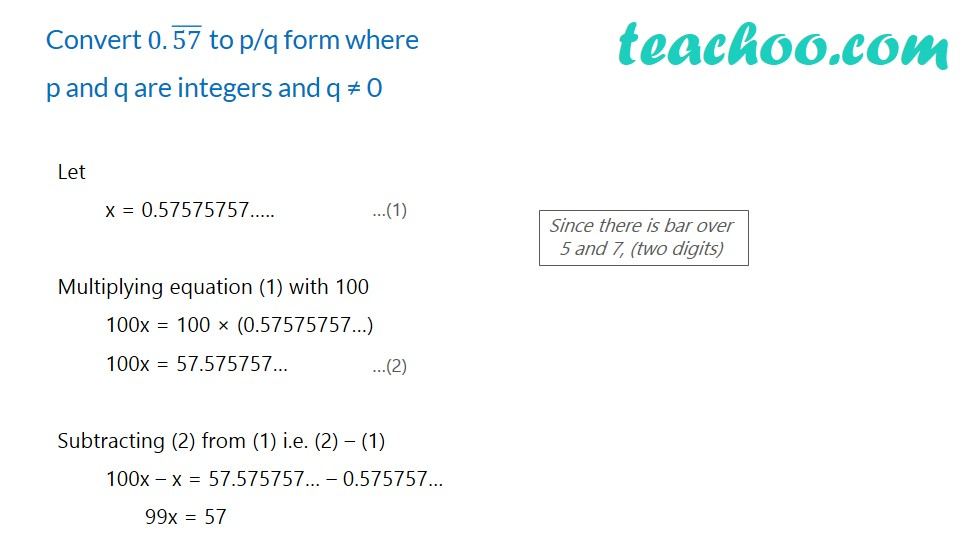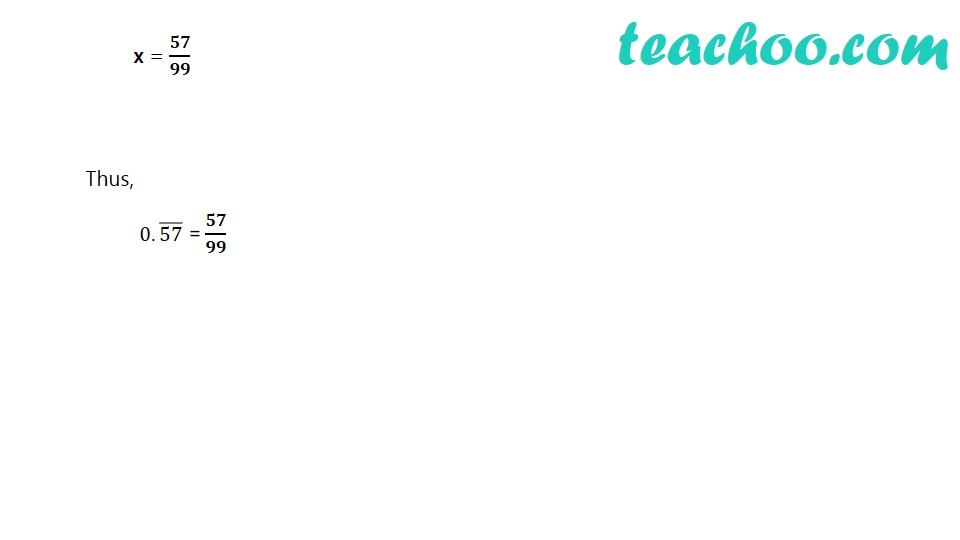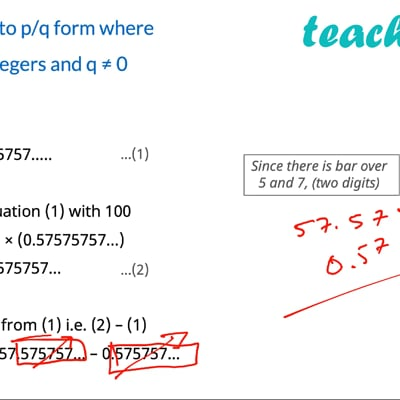Expressing decimal in p/q

Chapter 1 Class 9 Number Systems
Concept wiseThis video is only available for Teachoo black users

Introducing your new favourite teacher - Teachoo Black, at only ₹83 per month

### Transcript

Convert 0.(57) ̅ to p/q form where p and q are integers and q ≠ 0 Let x = 0.57575757….. Multiplying equation (1) with 100 100x = 100 × (0.57575757…) 100x = 57.575757… Subtracting (2) from (1) i.e. (2) – (1) 100x – x = 57.575757… – 0.575757… 99x = 57 Since there is bar over 5 and 7, (two digits) x = 𝟓𝟕/𝟗𝟗 Thus, 0.(57) ̅ = 𝟓𝟕/𝟗𝟗